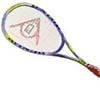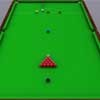#### You may also like### Rain or Shine

Predict future weather using the probability that tomorrow is wet given today is wet and the probability that tomorrow is wet given that today is dry.### Squash

If the score is 8-8 do I have more chance of winning if the winner is the first to reach 9 points or the first to reach 10 points?### Snooker

A player has probability 0.4 of winning a single game. What is his probability of winning a 'best of 15 games' tournament?

# Dicey Dice

##### Age 16 to 18Challenge Level

You can use common sense for simple comparisons between dice, but where the relative probabilities are not obvious you can use the formula for conditional expectation:

$$P(A> B) = P(A> B|A=1)P(A=1)+\dots + P(A> B|A=6)P(A=6)$$